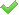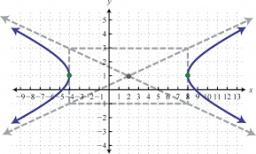# Hyperbola

Find the equation of hyperbola that passes through the point M [30; 24] and has focal points at F1 [0; 4 sqrt 6], F2 [0; -4 sqrt 6].

Result

h = (Correct answer is: )#### Solution:We would be pleased if you find an error in the word problem, spelling mistakes, or inaccuracies and send it to us. Thank you!Tips to related online calculators
Looking for help with calculating arithmetic mean?
For Basic calculations in analytic geometry is helpful line slope calculator. From coordinates of two points in the plane it calculate slope, normal and parametric line equation(s), slope, directional angle, direction vector, the length of segment, intersections the coordinate axes etc.
Looking for a statistical calculator?
Pythagorean theorem is the base for the right triangle calculator.Find the hyperbola equation with the center of S [0; 0], passing through the points: A [5; 3] B [8; -10]Solve for x: 7: x = 14: 1000